# Olympiad Test: Motion And Time

Test Description

## 15 Questions MCQ Test Science Olympiad Class 7 | Olympiad Test: Motion And Time

Olympiad Test: Motion And Time for Class 7 2023 is part of Science Olympiad Class 7 preparation. The Olympiad Test: Motion And Time questions and answers have been prepared according to the Class 7 exam syllabus.The Olympiad Test: Motion And Time MCQs are made for Class 7 2023 Exam. Find important definitions, questions, notes, meanings, examples, exercises, MCQs and online tests for Olympiad Test: Motion And Time below.
 1 Crore+ students have signed up on EduRev. Have you?
Olympiad Test: Motion And Time - Question 1

### If a body moves in a circle, it starts from point A and after completing the circle comes back to A; the displacement of such body is

Olympiad Test: Motion And Time - Question 2

### The CGS unit of speed is

Olympiad Test: Motion And Time - Question 3

### One mean solar day is equal to how many seconds?

Detailed Solution for Olympiad Test: Motion And Time - Question 3

Mean solar day = 24 h
= 24 × 60 × 60
= 86400 seconds

Olympiad Test: Motion And Time - Question 4

Which of the following graphs does not represent constant speed?

Detailed Solution for Olympiad Test: Motion And Time - Question 4

In a constant speed the change in position of the body remains the same for a particular interval of time. Break time axis into small parts and see the distance travelled in each of these parts is the same in choices A, B and C (zero in ‘C’). But the distance changes in ‘D’.

Olympiad Test: Motion And Time - Question 5

Which of the following is not in uniform motion?

Olympiad Test: Motion And Time - Question 6

Which of the following does not show oscillatory motion?

Olympiad Test: Motion And Time - Question 7

A jet is moving with a speed of 180 km/h. What is its speed in m/s?

Detailed Solution for Olympiad Test: Motion And Time - Question 7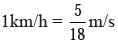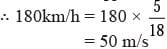Olympiad Test: Motion And Time - Question 8

Which of the following is the fastest?

Olympiad Test: Motion And Time - Question 9

Which of the following is matched incorrectly?

Detailed Solution for Olympiad Test: Motion And Time - Question 9

An odometer measures distances.

Olympiad Test: Motion And Time - Question 10

Distance travelled will be equal to the displacement if

Olympiad Test: Motion And Time - Question 11

The area of the shaded portion in the graph shown below represents?​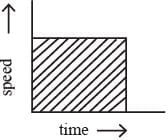Olympiad Test: Motion And Time - Question 12

The following graph shows the motion of four runners. P, Q, R and S in a 5 km marathon. Whose motion is the fastest?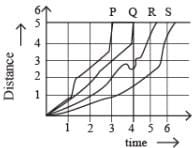Detailed Solution for Olympiad Test: Motion And Time - Question 12

The time taken by P is the lowest among the four, because more is the area under the wine, greater is the speed. Hence, P is the fastest.

Olympiad Test: Motion And Time - Question 13

With what speed should a car travel so that it can cover a distance of 5 km in 5 min?

Detailed Solution for Olympiad Test: Motion And Time - Question 13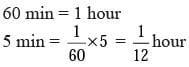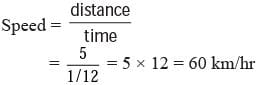Olympiad Test: Motion And Time - Question 14

All of the following are units time except.

Detailed Solution for Olympiad Test: Motion And Time - Question 14

A light year is the distance covered by light in one year, so it is the unit of distance and not of time.

Olympiad Test: Motion And Time - Question 15

Study the graph given below. How is the speed of the moving body?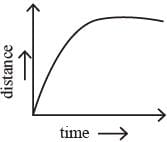Detailed Solution for Olympiad Test: Motion And Time - Question 15

Speed is the change of position of the body with respect to time. From the graph it can be seen that the change becomes less with time till there is no change at all i.e. till the speed becomes zero.